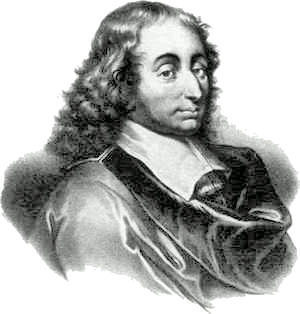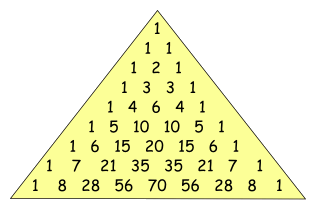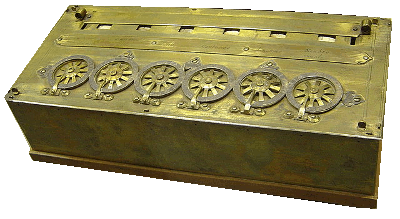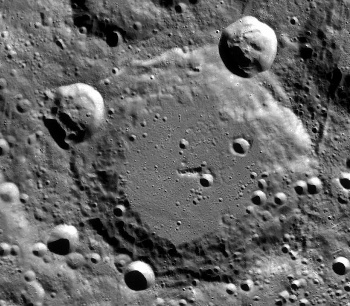### Blaise Pascal

June 19, 2012One important feature of science is that discoveries made centuries ago are still useful.

Today, June 19, is the anniversary of the birth of the
French mathematician, physicist, inventor and philosopher, Blaise Pascal (June 19, 1623 - August 19, 1662).

Although Pascal lived nearly four hundred years ago, his name is celebrated in many ways.Pascal's triangle, a triangular array of the binomial
coefficients.
Pascal's pyramid, the trinomial version of his triangle.
Pascal's theorem, a theorem about hexagons inscribed in conic
sections that he created when he was just sixteen.
• The pascal, a unit of pressure.
• The Pascal computer language.
Pascal's law of hydrostatics (ΔP = ρgΔh)
Pascal, a lunar crater at the northwest limb of the Moon.
Pascal's wager
As I wrote in a previous article (Extreme Intelligence, October 14, 2011) Pascal was a child prodigy who had an estimated intelligence quotient (IQ) of 195. As I wrote in that same article, Albert Einstein and Stephen Hawking are tied with estimated IQs of 160; while Richard Feynman had a measured IQ of "only" 126.

Mathematics

Pascal's triangle, introduced in his 1654 Traité du triangle arithmétique (Treatise on the Arithmetical Triangle) is known to most high school mathematics students. It's easy to construct, and it's a simple way to remember the coefficients of a binomial expansion; viz.,
(x + y)4 = x4 + 4x3y + 6x2y2 + 4xy3 + y4Nine rows of Pascal's triangle.

The fifth row gives the coefficients of the fourth power expansion, above.

(Rendered by the author using Inkscape)

Pascal is best known in mathematics for his work on probability theory. Pascal and Pierre de Fermat started the study of probabilities in an exchange of letters in 1654. They were inspired by the question of how many times a pair of dice must be cast before a double six will show. The answer, of course, is (6 x 6) = 36.

Some other of Pascal's triumphs involved the cycloid, the curve formed by a point at the perimeter of a rolling wheel (see figure). In 1634, Gilles de Roberval found that the area under a whole segment of the cycloid is 3πr2.(Modified Wikimedia Commons image))

Pascal extended this result by finding the area under any partial segment of the cycloid, and the volume and surface area of the solid of revolution formed by the rotation of the cycloid about the x-axis. These were major feats, since calculus was not available at his time.

Hydrostatics

There's good reason that the unit of pressure, the pascal, which is a newton's force acting over a square meter, is named after him. Pascal conducted some critical experiments in hydrostatics, including his famous barrel experiment, in which he demonstrated the pressure exerted by a long, thin column of water. Pascal was able to burst a wooden barrel through pressure exerted by water in a ten meter pipe attached to it.

This experiment confirmed his principle that pressure exerted anywhere in a confined incompressible fluid is transmitted equally throughout the fluid. His observations are summarized in his law of hydrostatics,
ΔP = ρgΔh
where ΔP is the pressure change in an incompressible fluid that results from a change in its height Δh. The constant ρ is the fluid density, and g is the gravitational acceleration.

Inventions

In a previous article (Who Invented the Computer?, March 21, 2011), I wrote about a prized possession of mine when I was a child. This was a plastic pencil box with a mechanical calculator built into its sliding door. A pencil point could be used to rotate a row of gear-like wheels to add and subtract very large numbers.

Pascal invented this mechanical calculator, called a Pascaline, in 1642 (see photograph). Pascal invented this to help his father, who was a tax collector, do calculations. The mechanism was much more complicated than the decimal calculator in my pencil box, since French currency at the time had 20 sols in a livre and 12 deniers in a sol. In the decade since its invention, fifty of these machines had been built, but few were sold.Blaise Pascal's Pascaline (1652). This unit, signed by Pascal, is in the Musée des arts et métiers, Paris.

(Photo by David Monniaux, via Wikimedia Commons)

Pascal, who was interested in the gaming arts from his work on probability, designed a roulette machine. It's written that this was an outgrowth of an attempt to build a perpetual motion machine.

Other Things Pascal

Many years ago I programmed extensively in Pascal, a language designed by famed computer scientist, Niklaus Wirth. This language was named in honor of Pascal, and Wirth cautioned everyone not to write it as PASCAL. I used this language on a VAX-11/780, and then migrated to personal computer versions when they became fast enough. Today, I write mostly in C.

There's a fairly large lunar crater (115 km diameter), named Pascal (see photograph).Image of Pascal crater (Coordinates: 74.6°N 70.3°W), taken on December 12, 2011, by the Lunar Reconnaissance Orbiter.

North is towards the upper right corner.

(NASA/LRO-LROC team image))

Pascal is known to non-scientists for his wager. Pascal reasoned through probability theory that it's only logical to believe in God. A person will lose nothing by believing in God, if God doesn't exist; whereas a person will lose everything by not believing, if God does exist.

There are many memorable quotations of Blaise Pascal, but I've found the following two to be the most interesting:
"I would have written a shorter letter, but I did not have the time." (Lettres provinciales, Letter XVI, 1656)

"...it is rare that mathematicians are intuitive, and that men of intuition are mathematicians." (Pensées, 1669)

### References:

Linked Keywords: Blaise Pascal; scienc; French; mathematician; physicist; inventor; philosopher; Pascal's triangle; triangular; array; binomial; coefficient; Pascal's pyramid; trinomial; Pascal's theorem; hexagons; conic section; pascal; pressure; Pascal computer language; Pascal's law of hydrostatics; Pascal crater; Pascal's wager; child prodigy; intelligence quotient; IQ; Albert Einstein; Stephen Hawking; Richard Feynman; Traité du triangle arithmétique; high school; mathematics; coefficient; binomial expansion; Inkscape; probability theory; Pierre de Fermat; probability; dice; cycloid; curve; point; perimeter; wheel; Gilles de Roberval; area; Wikimedia Commons; volume; solid of revolution; rotation; x-axis; calculus; newton; square meter; hydrostatics; barrel experiment; barrel; pipe; incompressible; fluid; law of hydrostatics; density; gravitational acceleration; pencil box; mechanical calculator; gear; Pascaline; tax collector; decimal; currency; Musée des arts et métiers; Paris; David Monniaux; roulette; perpetual motion machine; Pascal programming language; programming language; computer scientist; Niklaus Wirth; VAX-11/780; personal computer; C programming language; lunar crater; kilometer; km; Lunar Reconnaissance Orbiter; NASA; LRO-LROC team; Pascal's Wager; God; quotation; Lettres provinciales; Pensées; University of Adelaide; eBooks.